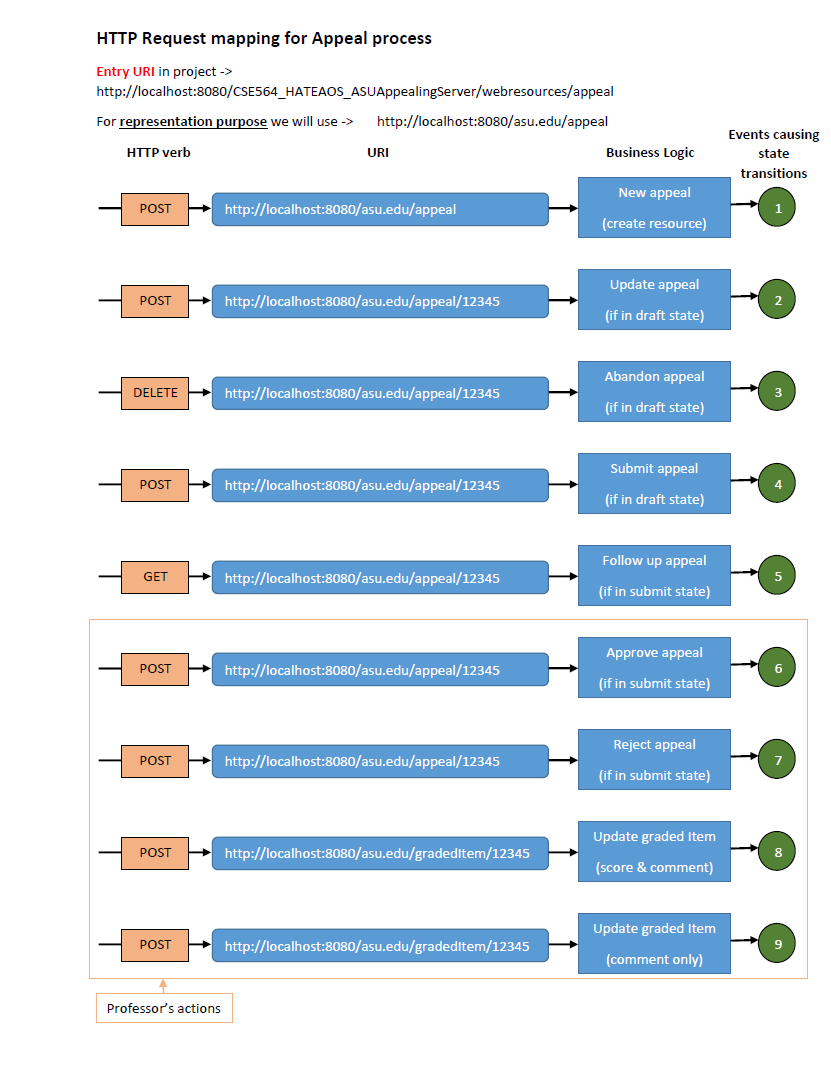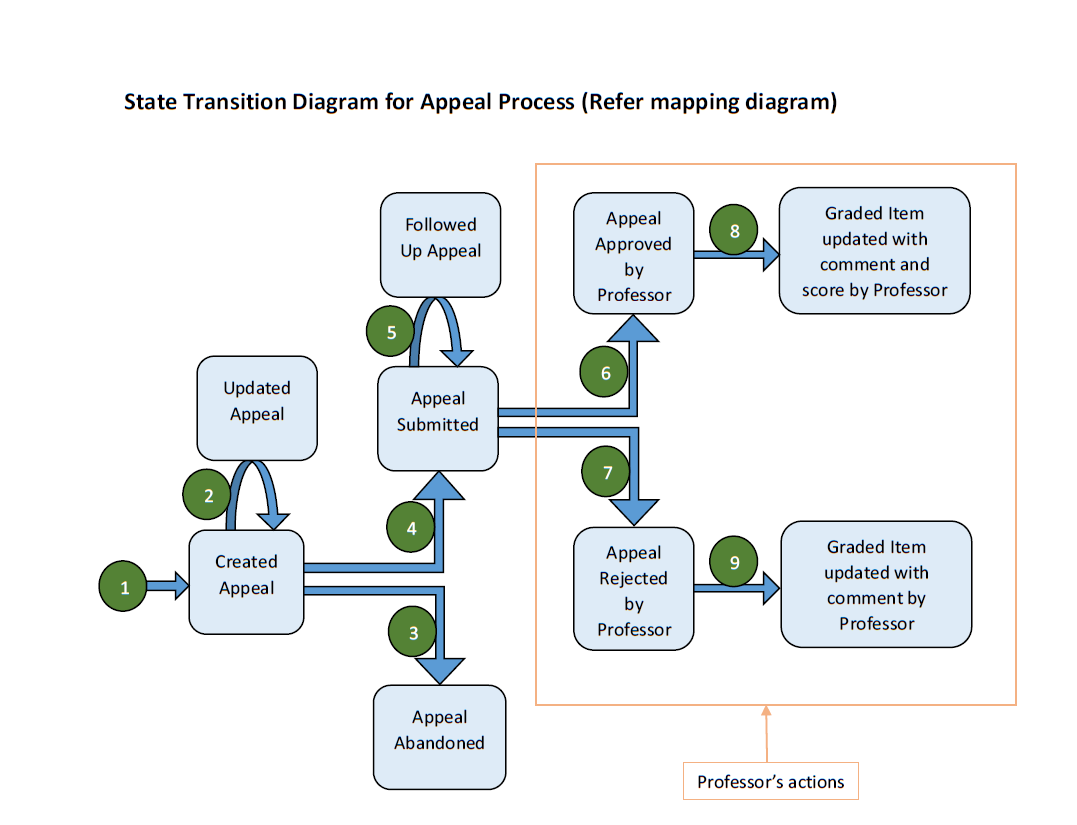View on GitHub

Java-portfolio

These are some challenging java programs and kind of awesome!

Welcome to Rajesh's Java Portfolio! Here is the list of some of the problem statement that I solved using Amazing Java!

1. Cracking the coding interview

This is a practice book on interview coding questions. I solved some of the problems from this book. Here is the list->

1. String Processing - View Code

• Implement an algorithm to determine if a string has all unique characters. What if you cannot use additional data structures?
• Given two strings, write a method to decide if one is a permutation of the other.
• Write a method to replace all spaces in a string with '%20'. You may assume that the string has sufficient space at the end of the string to hold the additional characters, and that you are given the "true" length of the string. (Note: if implementing in Java, please use a character array so that you can perform this operation in place.)
• Implement a method to perform basic string compression using the counts of repeated characters. For example, the string aabcccccaaa would become a2blc5a3. If the "compressed" string would not become smaller than the original string, your method should return the original string.
• Assume you have a method isSubstring which checks if one word is a substring of another. Given two strings, s1 and s2, write code to check if s2 is a rotation of s1 using only one call to isSubstring (e.g., "waterbottLe" is a rotation of "erbottLewat").

2. Hacker Rank

This is an online coding website. Here are some of the problems that I enjoyed solving->

1. Algorithm

These are the problems from algorithm section ->

• You are given a square matrix of size . Calculate the absolute difference of the sums across the two main diagonals. Code
• Your teacher has given you the task to draw the structure of a staircase. Being an expert programmer, you decided to make a program for the same. You are given the height of the staircase. You need to print a staircase as shown in the example. Code
• You are given time in AM/PM format. Convert this into a 24 hour format. Note Midnight is 12:00:00AM or 00:00:00 and 12 Noon is 12:00:00. Code

3. Miscellaneous

Coding practive around the internet.

1. Binary Tree View Code

• Returns true if given given target is in the binary tree.
• Insert given data into binary tree.
• Returns the number of nodes in the tree.
• Returns the max root-to-leaf depth of the tree.
• Returns the min value in a non-empty binary search tree.
• Returns the max value in a non-empty binary search tree.
• Prints the node values in the "inorder" order.
• Given a tree and a sum, returns true if there is a path from the root down to a leaf, such that adding up all the values along the path equals the given sum.
• Changes the tree into its mirror image.
• Changes the tree by inserting a duplicate node on each nodes's .left.
• Compares the receiver to another tree to see if they are structurally identical.
• Tests if a tree meets the conditions to be a binary search tree (BST).

2. Mixed Problems

• Given an array and target element, implement binary search. view code
• Given a number and its base, convert it to decimal number. view code
• Given graph and target element, search whether element presents in graph using depth-first search algorithm. view code
• Given number n, find nth number in Fibonacci sequence using recursion. view code
• Given two numbers, find GCD of those numbers using recursion. view code
• Given maze in the form of two dimensional array, find if there is a path from starting position 'S' to end position 'E'. view code
• Given set of elements, find power set of that set i.e. all combinations of elements from set. view code
• Given number, check if its prime. view code
• Given number n, find all prime numbers up to n using Sieve of Eratosthenes algorithm [fastest till date]. view code
• Assume that your language doesn't provide HashTable, implement one. view code

4. Creational Pattern view code

This project implements Singleton, Abstract Factory and Factory pattern.

Write a program that creates and prints out the gradebook for a class. The class consists of 2 kind of students: Graduate and Undergraduate and they have different grading criteria. The source feed for these 2 students is also in different formats. Graduate grades are store in an XML format and Undergraduate grades are in JSON format.

• Student Name

• Student ID

• Each Assigned work grouping with corresponding grades e.g., all Assignments together, all exams together, all quizzes together, …; in chronological order within the assigned work grouping

Note that he grade for an assigned work item can be numeric e.g., 88.5, or letter Grade e.g., A+. More grading notations are expected. To work out the final letter grade you need to get a numeric score. If the original grade was numeric then return the numeric grade, if the score is a letter grade then return the median of the spread for that letter grade. Use the class syllabus as the guide.

Implementation Constraints:

• You will need Factory Methods for reading in and outputting the Gradebook. You will also need a Factory Method for the Grade. More may be needed

• A class is either an undergraduate class or a graduate class, so you will need an Abstract Factory (a.k.a Kit) Pattern to implement the creational classes/objects for the different class gradebooks.

• Your program can exploit knowledge of the project’s file structure, but cannot assume knowledge on the directory structure outside the project. One thing this means is that you need to use relative addressing for the path to the input files rather than fixed addressing. If you hardcode in a path specific to you machine you can be sure it will fail on my machine.

How to run the project?

Please refer this document (click on 'View Raw') for instructions. [document]

Snapshot of application GUI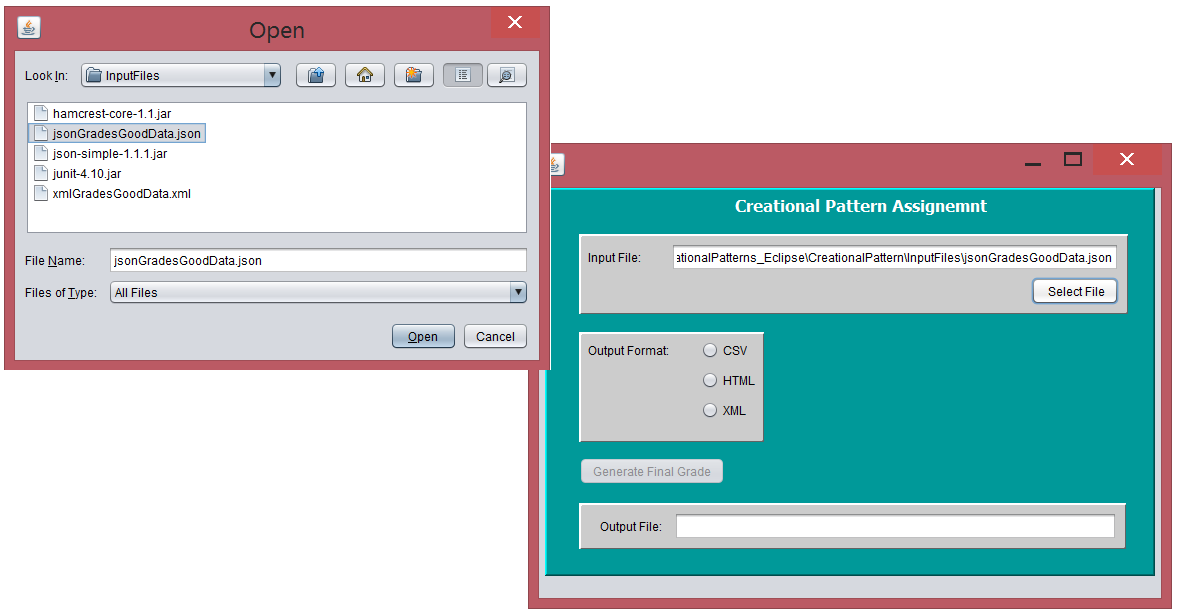5. Algorithm Design by Jon Kleinberg & Éva Tardos

Here is my attempt to convert some of the interesting algorithms into java code. I tried to make them as much simple and optimal as possible.

1. Greedy Algorithms [4th chapter]

We have a set of requests {1, 2 ..... n}; the ith request corresponds to an interval of time starting at s(i) and finishing at f(i). We’ll say that a subset of the requests is compatible if no two of them overlap in time, and our goal is to accept as large a compatible subset as possible. Compatible sets of maximum size will be called optimal.

If we have many identical resources available and we wish to schedule all the requests’ using as few resources as possible. Goal here is to partition all intervals across multiple resources.

2. Dynamic Programming [6th chapter]

The Weighted Interval Scheduling Problem is a strictly more general version, in which each interval has a certain value (or weight), and we want to accept a set of maximum value.

Consider a situation in which each item i has a nonnegative weight wi as before, and also a distinct value vi. Our goal is now to find a subset S of maximum value, subject to the restriction that the total weight of the set should not exceed W.

6. Threaded Design Pattern [view code]

This project implements Model-View-Controller, Intercepting Design Pattern.

Background: many companies and applications have rules in place with respect to input/output data. For example:

1. Call centers will often filter input messages for words they deem offensive

2. Companies will filter passwords to catch passwords that would be too easy to guess. E.g., a Disney employee using Disney character names for their password

3. Salting a password. This is the process of adding a known string to a user password to make it harder to break

Your assignment is to implement an MVC pattern that takes as input a password and will display the password and a message if it is valid or invalid according to a set of rules. You input screen should be form based and allow the user the select the password filter to apply and in what order. Your filter on the password check should use the Intercepting filter to insert the password checks between the Controller and the Model.

How to run the project?

Please refer this document for instructions. [document]

Snapshot of application GUI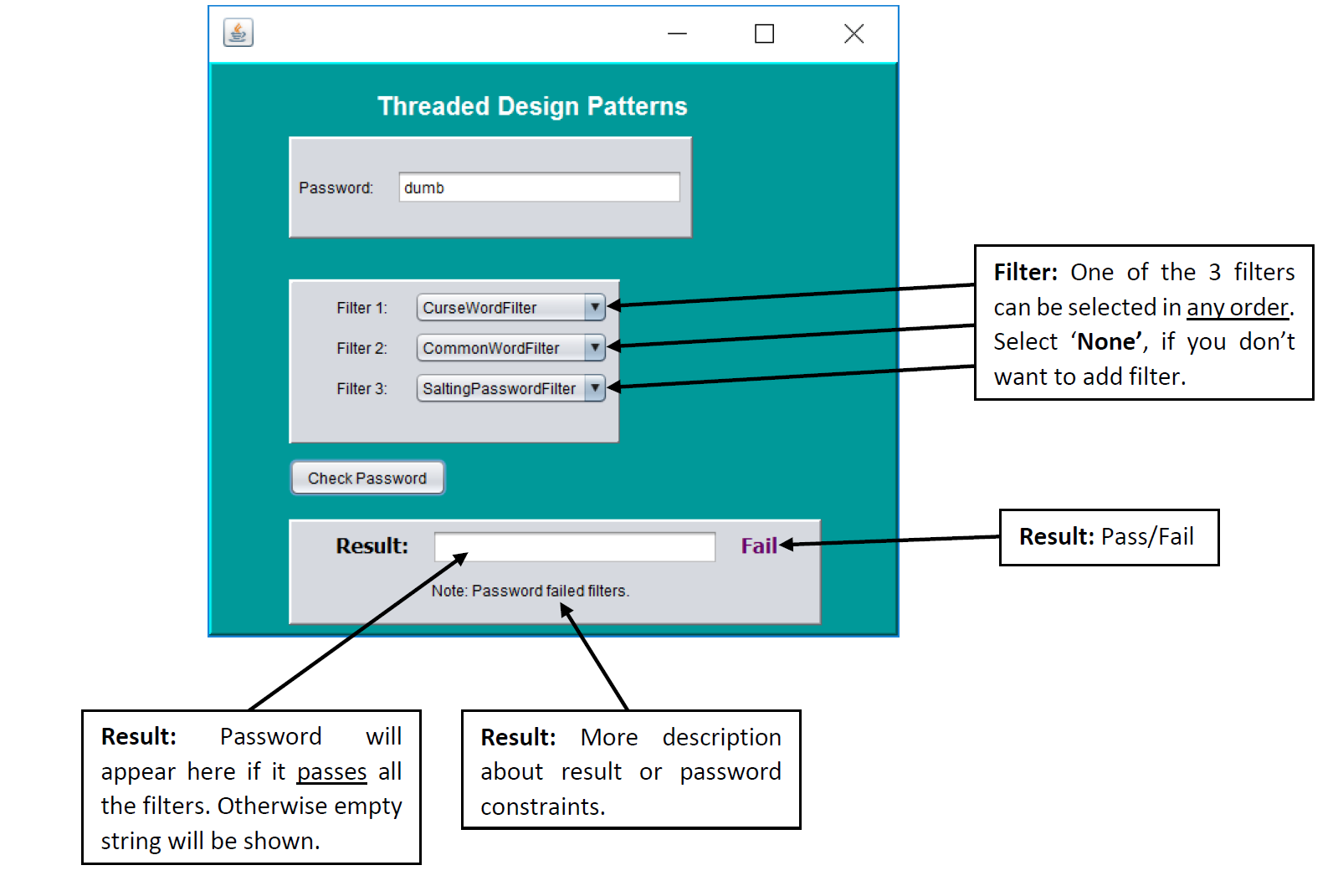7. LeetCode

Some of the challenges from www.leetcode.com

1. Contains Nearby Duplicate

Given an array of integers and an integer k, find out whether there are two distinct indices i and j in the array such that nums[i] = nums[j] and the difference between i and j is at most k. [View Code]

2. Delete Duplicates

Given a sorted linked list, delete all duplicates such that each element appear only once. [View Code]

3. Has Path Sum

Given a binary tree and a sum, determine if the tree has a root-to-leaf path such that adding up all the values along the path equals the given sum. [View Code]

4. Is Palindrome

Determine whether an integer is a palindrome. Do this without extra space. [View Code]

5. Is Same Tree

Given two binary trees, write a function to check if they are equal or not. Two binary trees are considered equal if they are structurally identical and the nodes have the same value. [View Code]

6. Largest Number Finder

Given a list of non negative integers, arrange them such that they form the largest number. For example, given [3, 30, 34, 5, 9], the largest formed number is 9534330. Note: The result may be very large, so you need to return a string instead of an integer. [View Code]

7. Max Depth of Binary Tree

Given a binary tree, find its maximum depth. The maximum depth is the number of nodes along the longest path from the root node down to the farthest leaf node.Given a binary tree, find its maximum depth. The maximum depth is the number of nodes along the longest path from the root node down to the farthest leaf node. [View Code]

8. Merge Two Lists

Merge two sorted linked lists and return it as a new list. The new list should be made by splicing together the nodes of the first two lists. [View Code]

9. Min Depth of Binary Tree

Given a binary tree, find its minimum depth. The minimum depth is the number of nodes along the shortest path from the root node down to the nearest leaf node. [View Code]

10. Min Stack

Design a stack that supports push, pop, top, and retrieving the minimum element in constant time. [View Code]

11. Move Zeros

Given an array nums, write a function to move all 0's to the end of it while maintaining the relative order of the non-zero elements. [View Code]

12. My Pow Function

Implement pow(x, n). [View Code]

13. Plus One

Given a non-negative number represented as an array of digits, plus one to the number. The digits are stored such that the most significant digit is at the head of the list. [View Code]

14. Reverse Integer

Reverse digits of an integer. [View Code]

15. Sort Colors

Given an array with n objects colored red, white or blue, sort them so that objects of the same color are adjacent, with the colors in the order red, white and blue. Here, we will use the integers 0, 1, and 2 to represent the color red, white, and blue respectively. [View Code]

16. Two sum

Given an array of integers, find two numbers such that they add up to a specific target number. The function twoSum should return indices of the two numbers such that they add up to the target, where index1 must be less than index2. Please note that your returned answers (both index1 and index2) are not zero-based. You may assume that each input would have exactly one solution. [View Code]

Input: numbers={2, 7, 11, 15}, target=9

Output: index1=1, index2=2

You are given two linked lists representing two non-negative numbers. The digits are stored in reverse order and each of their nodes contain a single digit. Add the two numbers and return it as a linked list. [View Code]

Input: (2 -> 4 -> 3) + (5 -> 6 -> 4)

Output: 7 -> 0 -> 8

18. Length of Longest Substring

Given a string, find the length of the longest substring without repeating characters. For example, the longest substring without repeating letters for "abcabcbb" is "abc", which the length is 3. For "bbbbb" the longest substring is "b", with the length of 1. [View Code]

8. Plain Old XML over HTTP [View Code]

In this project, client and server communicate using XML messages via HTTP. On client side I have provided interface to read Food Item data in XML/JSON format. I use Factory method and Singleton pattern to read XML and JSON data. There is also an interface to directly paste XML message which triggers the action on server side. Finally, I show up the XML message returned by the server.

On server side, I store the Food Item data in XML format. I used Jersey framework to provide Level-0 Restful services according to Richardson Maturity Model. Please refer documents in Info folder to run the project.

Here, is the client interface ->Client request and response ->9. RESTful-CRUD Service

In this project client and server communicate using HTTP verbs (POST, GET, PUT, DELETE) and JSON format for request/response body. Client interface allows professor to create, read, update, delete gradebook entries for given student. You can find the code here.

Gradebook structure is explained in following diagram->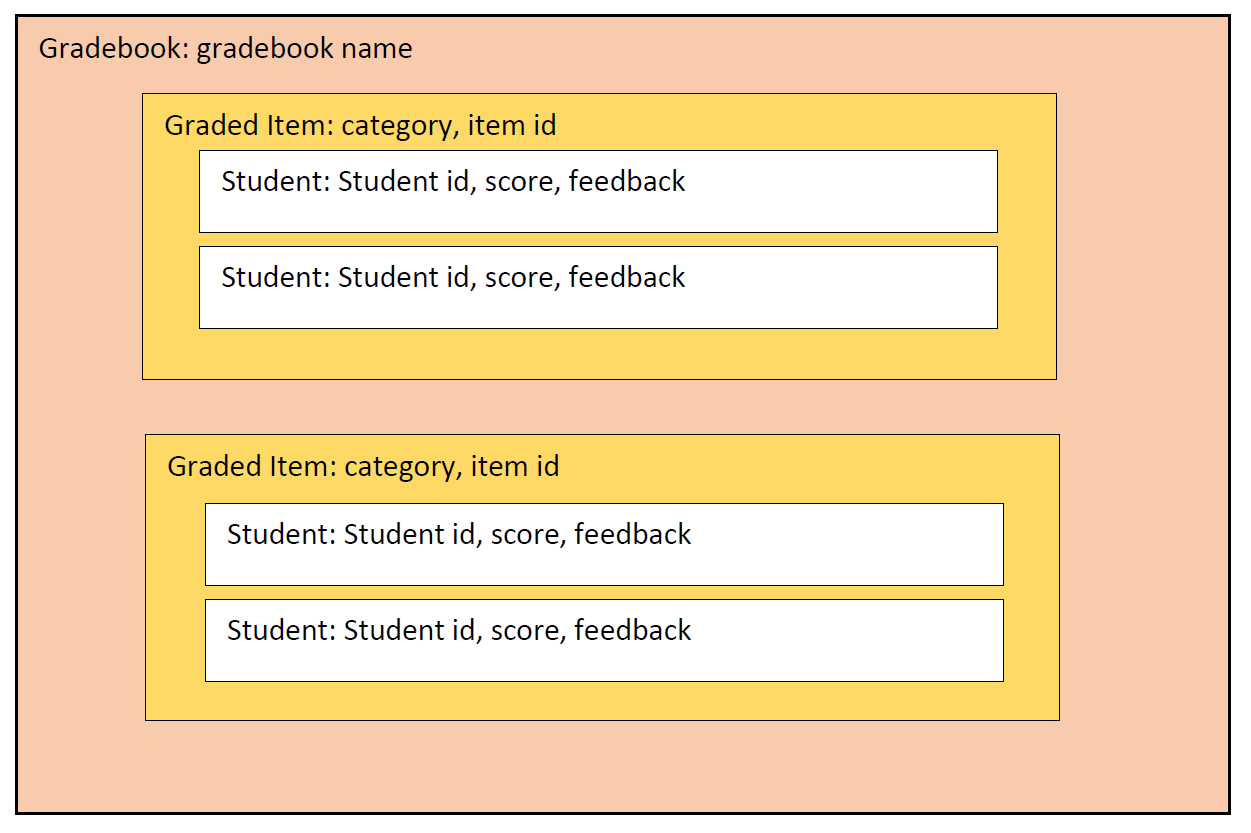Here is self explanatory image of client interface ->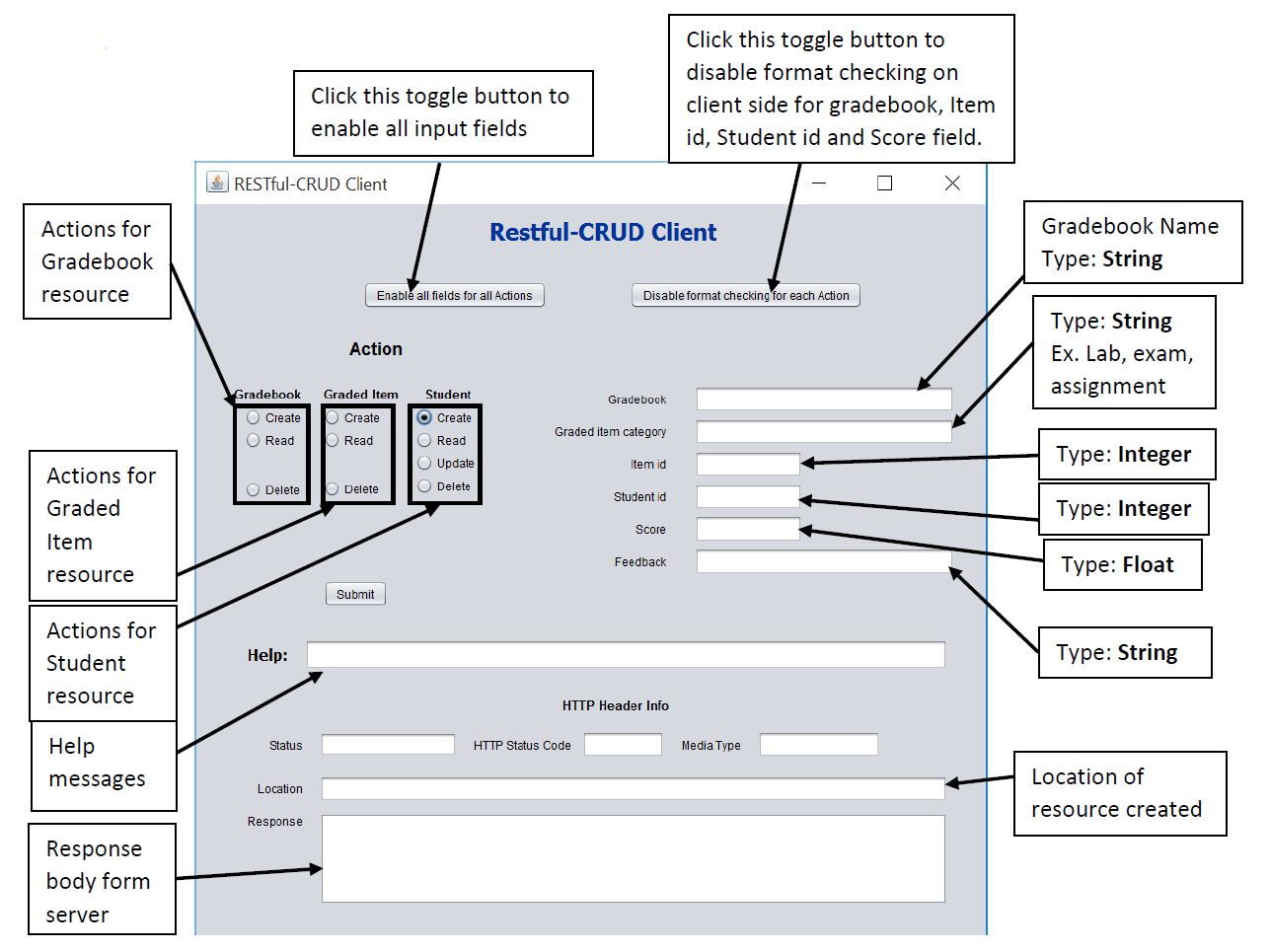URI for Student resource->

Some important points to know about project before you run it->

1. Client-main program is in edu.asu.cse564.samples.crud.restcl.ui.Gradebook_REST_UI.java
2. All client-server communication is done in JSON format.
3. Before you start creating graded items you need to create gradebook.
4. Server can maintain multiple gradebooks at a time. There could be multiple graded items inside gradebook. Graded Item contains multiple students’ info.
5. Item consists of category and Item id.
6. Before you start creating student you need to create graded item.
7. Student update action will update his score and feedback.
8. To access Student resource you need gradebook, category, Item id and Student id.
9. To access graded item resource you need gradebook, category, and Item id.
10. All gradebook data is saved in JSON file on server side. So, if you stop and start server it will be there.
11. You can see log statements while client and server are communicating to get idea what is happening behind the scene

10. HATEOAS RESTful Service

In this project, we implement the business logic using Hypermedia As The Engine Of Application State. According to Richardson's Maturity Model, this is the last level of Maturity of RESTful service. This should be the end-goal of any RESTful service. In this case, we only expose entry URI which will be permanent to client. Using Domain Application Protocol we guide the client to reach its goal using annotated Hypermedia links. For each action, Hypermedia representation tells the client what are the actions it can perform on resources and what the related resources are. This reduces the coupling between client and server. Except the entry URI, we can change any implementation on server-side any-time.